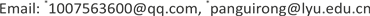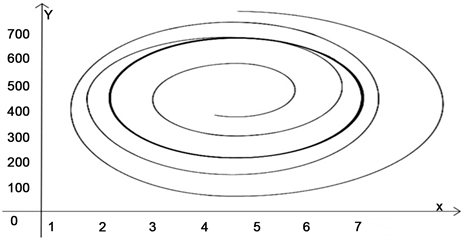﻿ 同质性物流企业竞争系统的动力学分析 Analysis of Dynamics of Competitive System of Homogeneous Logistics Enterprises

Advances in Applied Mathematics
Vol. 08  No. 06 ( 2019 ), Article ID: 30666 , 7 pages
10.12677/AAM.2019.86123

Analysis of Dynamics of Competitive System of Homogeneous Logistics Enterprises

Xia Li1*, Guirong Pan2*, Jia Li1, Wenya Jiang1

1School of Mathematics and Statistics, Linyi University, Linyi Shandong

2School of Information Science and Engineering, Linyi University, Linyi ShandongReceived: May 12th, 2019; accepted: May 30th, 2019; published: Jun. 6th, 2019ABSTRACT

In this paper, a dynamic model of regional logistics dynamics is established by using dynamic analysis method. Based on the qualitative analysis theory of differential equations, the existence and stability analysis of the equilibrium point and limit cycle of the system are carried out. The research results are verified by simulation method and the theoretical explanation is given. Finally, the research is applied to the development of logistics enterprises, which provides a feasible theoretical guidance for the development of regional logistics enterprises.

Keywords:Regional Logistics, Competition Model, Dynamics, Qualitative Analysis

1临沂大学数学与统计学院，山东 临沂

2临沂大学信息科学与工程学院，山东 临沂Copyright © 2019 by author(s) and Hans Publishers Inc.1. 引言

2. 区域物流动力学竞争模型

$\left\{\begin{array}{l}\frac{\text{d}x}{\text{d}t}={r}_{1}x\left(1-\frac{x}{P}\right)+{k}_{1}y\left(1-\frac{y}{Q}\right)-{l}_{1}xy\\ \frac{\text{d}y}{\text{d}t}={r}_{2}y\left(1-\frac{y}{Q}\right)+{k}_{2}x\left(1-\frac{x}{P}\right)-{l}_{2}xy\end{array}$ (2.1)

3. 模型分析

3.1. 平衡点分析

$\left\{\begin{array}{l}\frac{\text{d}x}{\text{d}t}={r}_{1}x+{k}_{1}y-\frac{{r}_{1}{x}^{2}}{P}-{l}_{1}xy-\frac{{k}_{1}}{Q}{y}^{2}\\ \frac{\text{d}y}{\text{d}t}={k}_{2}x+{r}_{2}y-\frac{{k}_{2}}{P}{x}^{2}-{l}_{2}xy-\frac{{r}_{2}{y}^{2}}{Q}\end{array}$ (3.1)

$\left\{\begin{array}{l}{r}_{1}x+{k}_{1}y-\frac{{r}_{1}{x}^{2}}{P}-{l}_{1}xy-\frac{{k}_{1}}{Q}{y}^{2}=0\\ {k}_{2}x+{r}_{2}y-\frac{{k}_{2}}{P}{x}^{2}-{l}_{2}xy-\frac{{r}_{2}{y}^{2}}{Q}=0\end{array}$

3.1.1. 考虑平衡点 $O\left(0,0\right)$

$\left\{\begin{array}{c}\frac{\text{d}x}{\text{d}t}={r}_{1}x+{k}_{1}y\\ \frac{\text{d}y}{\text{d}t}={k}_{2}x+{r}_{2}y\end{array}$ (3.2)

$\Delta ={\left({r}_{1}+{r}_{2}\right)}^{2}-4\left({r}_{1}{r}_{2}-{k}_{1}{k}_{2}\right)={\left({r}_{1}-{r}_{2}\right)}^{2}+4{k}_{1}{k}_{2}>0$ ，所以有两个实特征根：

${\lambda }_{1}=\frac{\left({r}_{1}+{r}_{2}\right)+\sqrt{\Delta }}{2}$${\mu }_{1}=\frac{\left({r}_{1}+{r}_{2}\right)-\sqrt{\Delta }}{2}$ ，显见， ${\lambda }_{1}>0$

1) 当 ${r}_{1}{r}_{2}-{k}_{1}{k}_{2}>0$ 时， ${r}_{1}+{r}_{2}>\sqrt{\Delta }$${\mu }_{1}>0$ ，此时， ${\lambda }_{1}$${\mu }_{1}$ 同号， $O\left(0,0\right)$ 为不稳定结点.

2) 当 ${r}_{1}{r}_{2}-{k}_{1}{k}_{2}<0$ 时， ${r}_{1}+{r}_{2}<\sqrt{\Delta }$${\mu }_{1}<0$ ，此时， ${\lambda }_{1}$${\mu }_{1}$ 异号， $O\left(0,0\right)$ 为鞍点。

3.1.2. 考虑平衡点 $A\left(P,0\right)$

$\left\{\begin{array}{l}\frac{\text{d}x}{\text{d}t}=-{r}_{1}x+\left({k}_{1}-{l}_{1}P\right)y-\frac{{r}_{1}}{P}{x}^{2}-{l}_{1}xy-\frac{{k}_{1}}{Q}{y}^{2}\\ \frac{\text{d}y}{\text{d}t}=-{k}_{2}x+\left({r}_{2}-{l}_{2}P\right)y-\frac{{k}_{2}}{P}{x}^{2}-{l}_{2}xy-\frac{{r}_{2}{y}^{2}}{Q}\end{array}$ (3.3)

${\lambda }^{2}+\left({r}_{1}-{r}_{2}+{l}_{2}P\right)\lambda -{r}_{1}\left({r}_{2}-{l}_{2}P\right)+{k}_{1}{k}_{2}-{k}_{2}{l}_{1}P=0$

$\Delta ={\left({r}_{1}-{r}_{2}+{l}_{2}P\right)}^{2}-4\left\{-{r}_{1}\left({r}_{2}-{l}_{2}P\right)+{k}_{1}{k}_{2}-{k}_{2}{l}_{1}P\right\}={\left({r}_{1}+{r}_{2}-{l}_{2}P\right)}^{2}-4{k}_{2}\left({k}_{1}-{l}_{1}P\right)$

1) 当 $\Delta >0$ 时，有两个实特征根： ${\lambda }_{2}=\frac{\left({r}_{2}-{r}_{1}-{l}_{2}P\right)+\sqrt{\Delta }}{2}$${\mu }_{2}=\frac{\left({r}_{2}-{r}_{1}-{l}_{2}P\right)-\sqrt{\Delta }}{2}$

a) 当 $|{r}_{2}-{r}_{1}-{l}_{2}P|>\sqrt{\Delta }$ 时， ${\lambda }_{2}$${\mu }_{2}$ 同号，所以 $A\left(P,0\right)$ 是结点。此时，若 ${r}_{2}-{r}_{1}-{l}_{2}P<0$$A\left(P,0\right)$ 是稳定结点， ${r}_{2}-{r}_{1}-{l}_{2}P>0$$A\left(P,0\right)$ 是不稳定结点。

b) 当 $|{r}_{2}-{r}_{1}-{l}_{2}P|<\sqrt{\Delta }$ 时， ${\lambda }_{2}$${\mu }_{2}$ 异号，所以 $A\left(P,0\right)$ 是鞍点。

2) 当 $\Delta <0$ 时，方程有共轭虚根： ${\lambda }_{2}=\frac{\left({r}_{2}-{r}_{1}-{l}_{2}P\right)+i\sqrt{-\Delta }}{2}$${\mu }_{2}=\frac{\left({r}_{2}-{r}_{1}-{l}_{2}P\right)-i\sqrt{-\Delta }}{2}$

3.1.3. 考虑平衡点 $B\left(0,Q\right)$

$\left\{\begin{array}{l}\frac{\text{d}x}{\text{d}t}=\left({r}_{1}-{l}_{1}Q\right)x-{k}_{1}y-\frac{{r}_{1}}{P}{x}^{2}-{l}_{1}xy-\frac{{k}_{1}}{Q}{y}^{2}\\ \frac{\text{d}y}{\text{d}t}=\left({k}_{2}-{l}_{2}Q\right)x-{r}_{2}y-\frac{{k}_{2}}{P}{x}^{2}-{l}_{2}xy-\frac{{r}_{2}}{Q}{y}^{2}\end{array}$ (3.4)

${\lambda }^{2}+\left({r}_{2}-{r}_{1}+{l}_{1}Q\right)\lambda -{r}_{2}\left({r}_{1}-{l}_{1}Q\right)+{k}_{1}{k}_{2}-{k}_{1}{l}_{2}Q=0$

$\Delta ={\left({r}_{1}+{r}_{2}-{l}_{1}Q\right)}^{2}-4{k}_{1}\left({k}_{2}-{l}_{2}Q\right)$

1) 当 $\Delta >0$ 时，有两个实特征根： ${\lambda }_{3}=\frac{\left({r}_{1}-{r}_{2}-{l}_{1}Q\right)+\sqrt{\Delta }}{2}$${\mu }_{3}=\frac{\left({r}_{1}-{r}_{2}-{l}_{1}Q\right)-\sqrt{\Delta }}{2}$

a) 当 $|{r}_{1}-{r}_{2}-{l}_{1}Q|>\sqrt{\Delta }$ 时， ${\lambda }_{3}$${\mu }_{3}$ 同号，所以 $B\left(0,Q\right)$ 是结点。此时，若 ${r}_{1}-{r}_{2}-{l}_{1}Q<0$$B\left(0,Q\right)$ 是稳定结点， ${r}_{1}-{r}_{2}-{l}_{1}Q>0$$B\left(0,Q\right)$ 是不稳定结点。

b) 当 $|{r}_{1}-{r}_{2}-{l}_{1}Q|<\sqrt{\Delta }$ 时， ${\lambda }_{3}$${\mu }_{3}$ 异号，所以 $B\left(0,Q\right)$ 是鞍点。

2) 当 $\Delta <0$ 时，方程有共轭虚根： ${\lambda }_{3}=\frac{\left({r}_{1}-{r}_{2}-{l}_{1}Q\right)+i\sqrt{-\Delta }}{2}$${\mu }_{3}=\frac{\left({r}_{1}-{r}_{2}-{l}_{1}Q\right)-i\sqrt{-\Delta }}{2}$

3.2. 极限环分析

$\left\{\begin{array}{l}\frac{\text{d}x}{\text{d}t}={r}_{1}x\left(1-\frac{{x}^{2}}{P}\right)-{k}_{1}y-{l}_{1}x{y}^{2}\\ \frac{\text{d}y}{\text{d}t}={r}_{2}y\left(1-\frac{{y}^{2}}{Q}\right)+{k}_{2}x-{l}_{2}{x}^{2}y\end{array}$ ，即 $\left\{\begin{array}{l}\frac{\text{d}x}{\text{d}t}={r}_{1}x-{k}_{1}y-x\left(\frac{{r}_{1}{x}^{2}}{P}+{l}_{1}{y}^{2}\right)\\ \frac{\text{d}y}{\text{d}t}={k}_{2}x+{r}_{2}y-y\left({l}_{2}{x}^{2}+\frac{{r}_{2}{y}^{2}}{Q}\right)\end{array}$

$\left\{\begin{array}{l}\frac{\text{d}x}{\text{d}t}=Mx-ky-mx\left({x}^{2}+{y}^{2}\right)\\ \frac{\text{d}y}{\text{d}t}=My+kx-my\left({x}^{2}+{y}^{2}\right)\end{array}$ (3.5)

$\left\{\begin{array}{l}\frac{\text{d}x}{\text{d}t}=-ky-mx\left({x}^{2}+{y}^{2}-\frac{M}{m}\right)\\ \frac{\text{d}y}{\text{d}t}=kx-my\left({x}^{2}+{y}^{2}-\frac{M}{m}\right)\end{array}$ (3.6)

$\left\{\begin{array}{l}\frac{\text{d}r}{\text{d}t}=-mr\left({r}^{2}-\frac{M}{m}\right)\\ \frac{\text{d}\theta }{\text{d}t}=k\end{array}$ (3.7)

$r={r}_{1}<\sqrt{\frac{M}{m}}$ 时， $\frac{\text{d}r}{\text{d}t}=-m{r}_{1}\left({r}_{1}^{2}-\frac{M}{m}\right)>0$ ，r是t的递增函数，因此，随着t的增大，轨线从圆 $r={r}_{1}$ 上走出圆外。

$r={r}_{2}>\sqrt{\frac{M}{m}}$ 时， $\frac{\text{d}r}{\text{d}t}=-m{r}_{2}\left({r}_{2}^{2}-\frac{M}{m}\right)<0$ ，r是t的递减函数，因此，随着t的增大，轨线从圆 $r={r}_{2}$ 上走进圆内。Figure 1. Distribution phase diagram of the inside and outside orbits of the limit cycle

4. 结论

Analysis of Dynamics of Competitive System of Homogeneous Logistics Enterprises[J]. 应用数学进展, 2019, 08(06): 1072-1078. https://doi.org/10.12677/AAM.2019.86123

1. 1. 杨波, 薛伟. 区于物流系统动力学方针研究[J]. 森林工程, 2009, 25(10): 81-85.

2. 2. 李丽萍. 区域物流系统动力学模型构建与应用研究[D]: [博士学位论文]. 哈尔滨: 东北林业大学, 2012.

3. 3. 张文杰. 区域经济发展与物流[J]. 物流技术, 2002(3): 7-9.

4. 4. 王树禾. 微分方程模型与混沌[M]. 合肥: 中国科学技术大学出版社, 1999.

5. 5. 姜启源, 谢金星, 叶俊. 数学模型[M]. 北京: 高等教育出版社, 2018.

6. 6. 丁同仁. 常微分方程教程[M]. 北京: 人民教育出版社, 1963.

7. NOTES

*通讯作者。